• whizible
• October 1, 2018

The concept of Earned Schedule (ES) is similar to and actually derived from Earned Value.  However, Earned Schedule uses time units for measuring schedule performance instead of cost units. It was developed by Walt Lipke in 2003. In PMI’s Practice Standard for EVM, Second Edition (2011), a complete coverage of earned schedule can be found in Appendix D. Earned Schedule is also included in the PMI PMBOK (6th edition) released in 2017.

Earned Schedule (ES) can be considered as an extension to Earned Value Methodology (EVM). No additional data is needed for computing the ES values; only the data from EVM is required. Measuring schedule performance in time units (using ES) is more intuitive than measuring it in cost units (using EVM for cost); for instance, it is more natural to say that a project is behind schedule by x weeks than by y dollars. There are a number of benefits of using ES, the main being:

1. To overcome the quirky nature of Schedule Variance (SV) and Schedule Performance Indicator (SPI). When using cost based EVM, as we approach end of project, SV goes to zero and SPI to unity (1), implying perfect schedule performance. This is counter intuitive in case of projects finishing late. On the other hand, Earned Schedule based indicators, SV(t) and SPI(t) (suffix ‘t ‘ indicates that they are time based rather than cost based) behave appropriately and consistently throughout the entire period of project performance. SV(t) is negative and SPI(t) less than 1.0 for a project behind schedule. SV(t) will only revert to zero and SPI(t) to 1 when project completes on time, not otherwise.
2. Ability to more accurately predict the completion dates for projects that are behind schedule.
3. As a bridge for performing meaningful schedule analysis.

# Understanding Earned Schedule (ES)

Conceptually, ES can be calculated by projecting EV at status duration onto PV curve and then taking X-intercept of this projection, where time period (in weeks or months) is shown on X-axis.

This can be typically depicted in an S-curve diagram like the one shown below. The cumulative amounts of Earned Value (EVcum) and Planned Value (PVcum) are shown on Y-axis, while time periods are on X-axis. Here, status is taken at period #8. By projecting the point on EV curve at \$1900 to PV curve and then tracing the point from PV curve to X-axis, we can determine amount of Earned Schedule in time units (in the figure, ES is  @7.3 wk, and schedule variance is  negative 0.7 weeks)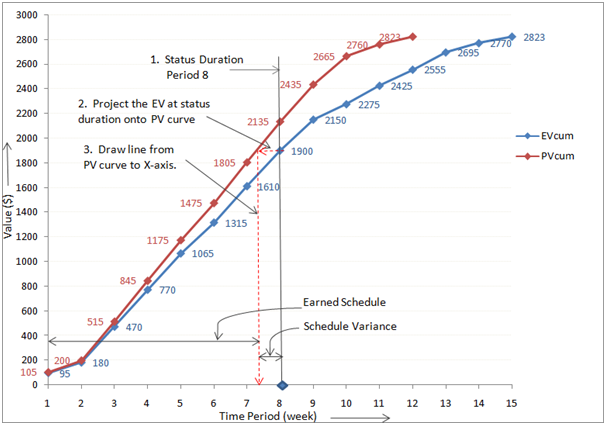In the example above, Planned Duration (PD) for the project was 12 weeks, while actual duration was 15 weeks. Thus the project finished late and was behind schedule when complete.

Earned Schedule can be formally computed using the expression below:

EScum = C + I

where, C is the number of time periods for which EV >= PV, and,

I is the fraction of PV increment calculated as linear interpolation,

I = (EV – PVc) / (PVc+1 – PVc)

where,  PVc = PV corresponding to time period C,

PVc+1 = PV corresponding to time period C+1

To make the ES calculation process more intuitive and accurate, we can visualize the EV & PV data on a stacked bar diagram. The cumulative amounts of EV and PV are shown as two stacked bars, with labels for all time periods. The PV bar has 12 time periods corresponding to PD of 12 weeks, whereas EV bar shows 15.

ES can be easily computed for any time period by projecting the EV value for that period from the top bar (EV) onto the bottom bar (PV).

As shown in the diagram, EV = 1900 for time period #8.

We can see that at this point, EV accrued is more than 7 complete periods on the PV bar at bottom. So the value of C in the ES equation above is 7. To calculate I, which is the PV increment earned, we simply substitute corresponding EV & PV values for the periods of 7 & 8. Thus,

I = (1900 – 1805) / (2135 – 1805) = 95/330 = 0.288

Therefore, ES = C + I = 7 + 0.288 = 7.288 wk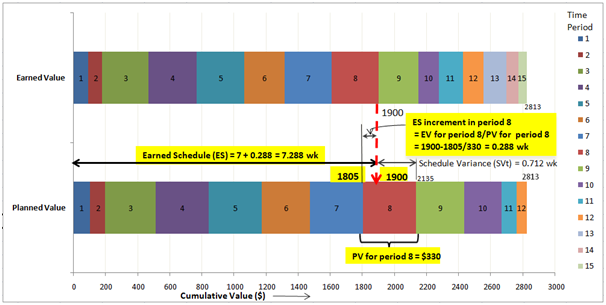we can calculate periodic & cumulative measures of ES and plot on graphs, as shown below.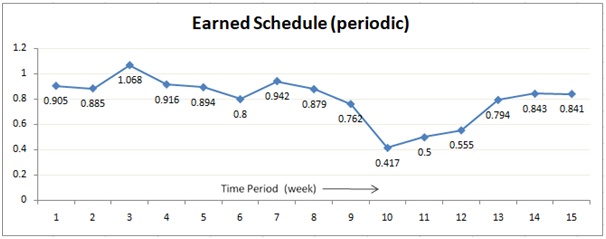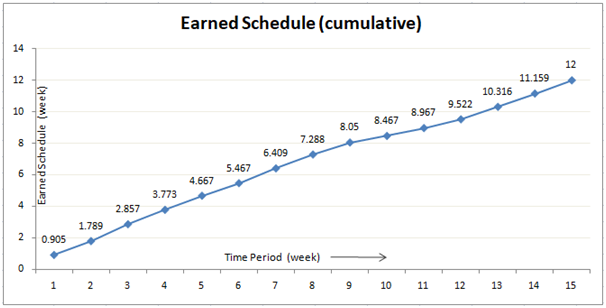An important indicator, time based Schedule Performance Index (SPIt) can be calculated using ES as the ratio of ES to actual time (AT) in weeks or months at the status duration. It indicates how efficiently the available time is being utilized for project work.

SPI(t)  =  ES / AT

where AT is the actual time.

So SPI(t) is a measure of time-based schedule efficiency. When SPI(t) >= 1, it indicates favourable & efficient schedule performance while SPIt < 1 indicates unfavourable project condition.

In our example, we can calculate SPI(t) for all time periods and plot the values on a graph. As can be seen from the graph, SPI(t) value has been continuously below 1 which indicates poor schedule performance. Peak efficiency of @95% was achieved in period #3, it fell continually afterwards.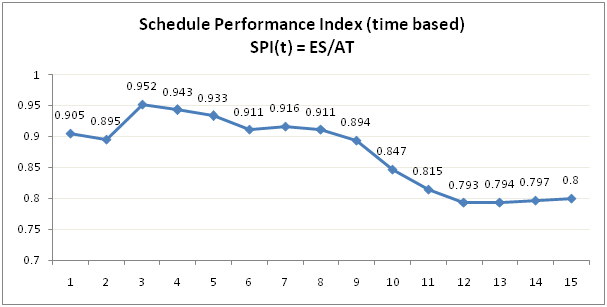One of the most important benefits of using ES is the ability to predict completion time. We can calculate Independent Estimate At Completion-time based (IEAC(t)) which is a statistical estimate of total time for completing project work. It can be calculated using the most commonly used formula:

IEAC(t) = PD / SPI(t)   —————————- (1)

where,  PD is the Planned duration in time units

If we use a customized Performance Factor (PFt) instead of SPIt, then the equation is:

IEAC(t)  =  AT + (PD – ES)/PF(t) —————— (2)

where, AT is the Actual Time in time units at status date

PF(t) is Performance Factor(time based) which allows to predict completion time more accurately. When PF(t) = SPI(t), equation (2) is equivalent to equation (1)

Using IEAC(t), Independent Estimate for project Completion Date (IECD) can be easily calculated as:

IECD = Project Start Date + IEAC(t)

By adding trend lines to IEAC(t) graph as shown below, one can get early indications of completion time as compared to baseline schedule. In the diagram, an early trend line at the end of Period #2 predicts project duration of @15 weeks or a delay of @3 weeks, while an intermediate trend curve drawn at period #6 projects to a duration of @16 weeks or a delay of @4 weeks compared to the planned duration of 12 weeks.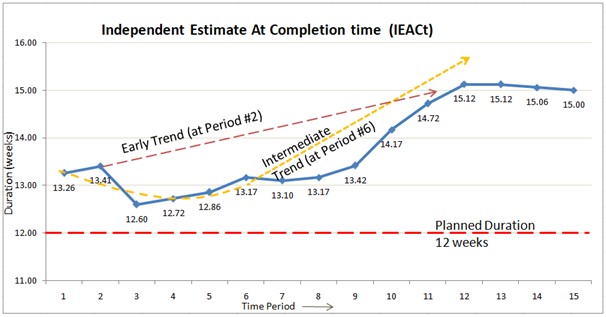ES concept provides another key indicator called “To complete” Schedule Performance Index (TSPI), which is similar to the metric TCPI (To Complete Performance Index) available with EVM for cost. TSPI forecasts future performance required by a project team to bring the behind schedule project back on track. TSPI can be determined as:

TSPI = (PD-ES) / (PD-AT) and

TSPI = (PD-ES) / (ED-AT),  where ED is the project manager’s estimated completion duration.

TSPI value above 1 indicates that the project team needs to work harder to utilize remaining time, while a value of below 1 indicates that even a bit below par performance may achieve the target schedule. Based on research, when TSPI is greater than 1.1, the projected duration (PD or ED) is likely to be unachievable. A value less than 1.1 suggests that schedule delay may be recoverable if timely and corrective action is planned.

As shown by the graph below, by Period #6 when TSPI is 1.09, it is pretty clear that achieving original schedule of 12 weeks is quite difficult. The project manager has a few options such as having the project team work at @110% efficiency for the remaining time, for instance doing a 9 hr task in 8 hours, or re-estimating and re-baselining to increase project duration (which will also increase cost).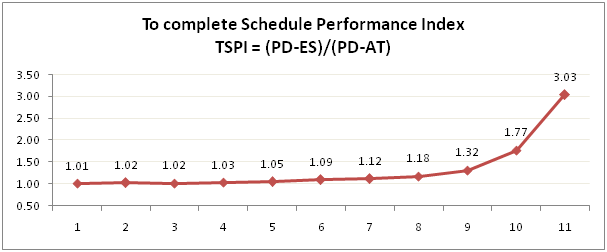If a project or task is overdue, we cannot forecast the performance needed to bring it back on track or TSPI is not applicable. In such a case, the project manager needs the best performance from the team to minimize schedule variance. Time based Schedule Variance (SVt) can be simply calculated as difference between ES and AT. When SVt is positive, it indicates that project is ahead of schedule; when it is negative as shown here, it means project is behind schedule. As seen here, the project finished with SV(t) value of -3 week or 3 weeks behind schedule compared to the original schedule of 12 weeks. Project manager should always monitor SVt and take corrective action to minimize it.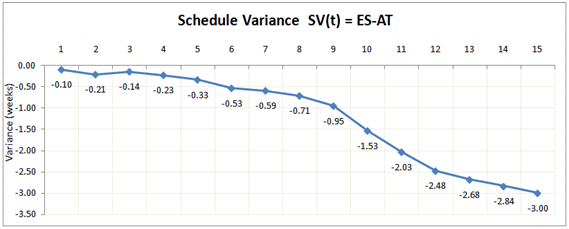EVM data (PV, EV, AC, etc.) can be imported or entered to get deeper insights into the health of the project than traditional EVM analysis with Whizible EVM/ES Analysis. Whizible’s upcoming EVM/ES Analytics tool can be used at project and/or task level where EVM data is available. Summary data such as periodic & cumulative ES, SV(t); indicators such as SPI(t) & TSPI;  estimates like IEAC & IECD, and charts are available to be made part of periodic monitoring and reports. With these charts & indices, project managers can see trends in the estimated completion date and cost, and decide when re-baselining may be appropriate.

### About the author

Shailesh Joshi works as Project Management Consultant for new product initiatives at Whizible.
He is leading efforts for brand-new User Experience and introducing smart, intelligent features across Whizible’s portfolio of Project & Service Automation tools.
Shailesh has more than 20 years of experience in Technology and Management. He began his career at ComputerVision Systems (now Parametric Technology Corp.) where he held development and leadership positions. After working in consulting roles at Fortune companies such as Equifax, Home Depot, UPS and USBancorp, he led development of virtual Queue Management product for a start-up company Quezone.
Shailesh holds bachelor’s degree in Engineering from University of Mumbai and is PMI certified Project Management Professional (PMP).

Subscribe to Whizible Blog